# Time functions?

I have two columns (`starting`,`ending`) with time values (`07:45:00`,`16:15:00`). Is there a way to calculate the difference in full or partial hours?

Hi Craig,

The following formula should work for you:

``````if not \$starting or not \$ending:
return None
s = (\$starting - \$ending).total_seconds()
hours = divmod(abs(s), 3600)
minutes = divmod(hours, 60)
seconds = divmod(minutes, 1)
return "%dhr %dmin %ds" % (hours, minutes, seconds)
``````

Natalie-

Here’s an example of the data (`Hours` is manually entered):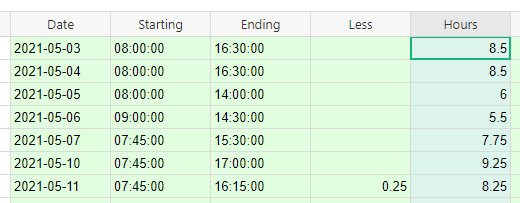As you can see, the two times are text values.

As a result, I get an error when attempting to subtract them:

``````TypeError: unsupported operand type(s) for -: 'str' and 'str'
``````

What’s your recommendation for storing times?

Thanks.

We recommend storing times using the DateTime column type like you see in the screenshot below. You can choose from different Date and Time formats but you can also create custom formats for each.

When you convert the column to DateTime, it does put the time you have entered into the space for date. If you just have 10 or so rows, you can copy the time from this spot, select the date then paste the time into the spot on the right. If you have a lot of rows, this is time consuming and I have another method below for you.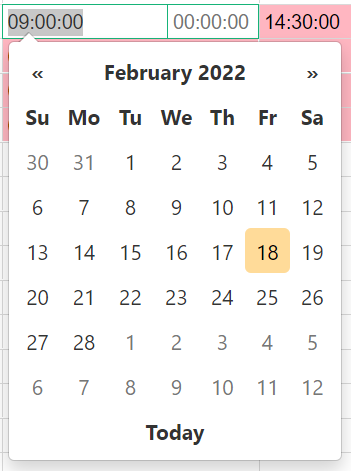We can use python to combine the Date and Starting column to get it into our datetime format. Add a new row to the right of Starting. I’ve named mine datetime Starting. Enter the formula below:

``````str(\$Date)+" "+\$Starting
``````

str() converts the date into a string format. This is assuming the Date column is using the Date column Type. If it is not, you can remove str() from the equation so it’s just `\$Date+" "+\$Starting`

This is what you should see: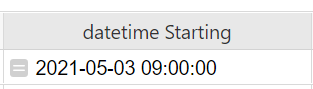Convert the datetime Starting column to the datetime column type and set timezone as well as the date and time formats to whatever format you prefer.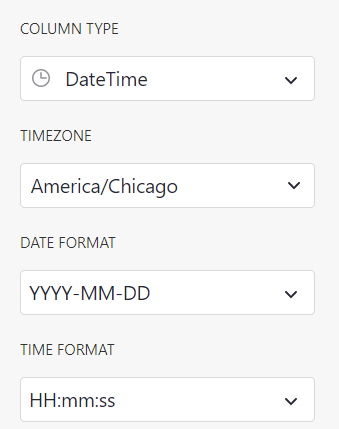Then, you will do this again for the Ending time. Create a new column and enter the following formula:

``````str(\$Date)+" "+\$Ending
``````

Then, convert to datetime and select your formatting preferences.

Because these two new columns are formula columns, you’d be required to keep all date, time and datetime columns. If you wish to only have Starting and Ending using the datetime format, copy/paste the values from the new datetime Starting and Ending columns into new columns. You can select the entire column by selecting the column header.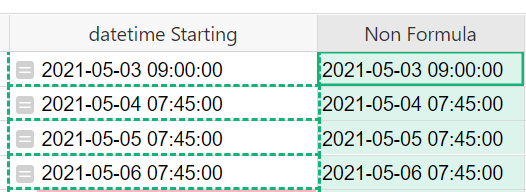Once you have your two new datetime columns, you’ll be able to use the formula to calculate the difference in times.

``````if not \$starting or not \$ending:
return None
s = (\$starting - \$ending).total_seconds()
hours = divmod(abs(s), 3600)
minutes = divmod(hours, 60)
seconds = divmod(minutes, 1)
return "%dhr %dmin %ds" % (hours, minutes, seconds)
``````

Be sure to update \$starting and \$ending with the final column names for the datetime columns and keep in mind, capitalization matters so if the column names are Starting and Ending, be sure they are in the formula as \$Starting and \$Ending.

Thanks for the help.

1 Like